# イメージキャプショニング入門

#### 実装

from keras.layers import Input, Dense, LSTM, Embedding, Dropout
from keras.layers.merge import concatenate

def define_model(vocab_size, max_length):
#photo feature extractor
inputs1 = Input(shape=(2048, ))
fe1 = Dropout(0.5)(inputs1)
fe2 = Dense(256, activation='relu')(fe1)

#sequence model
inputs2 = Input(shape=(max_length, ))
se2 = Dropout(0.5)(se1)
se3 = LSTM(256)(se2)

#decoder model
decoder1 = concatenate([fe2, se3])
decoder2 = Dense(256, activation='relu')(decoder1)
outputs = Dense(vocab_size, activation='softmax')(decoder2)

model = Model(inputs=[inputs1, inputs2], outputs=outputs)

return model


#### まとめ

イメージキャプショニングの大まかな構成と流れについて見てきました。画像(や動画)と言語が交わる分野には、イメージキャプショニングの他にもビデオキャプショニング、動画のアクション理解、ビジュアル質問応答、映像要約など、保育の世界でも役に立つ可能性がある技術がたくさんあります。引き続き注目していきたいと思います。

# 基本的なサンプリングアルゴリズムである棄却サンプリングを試してみた

#### はじめに

こんにちわ、研究開発部の島田です。今回は統計的学習で基本的なサンプリングアルゴリズムを一つ紹介します。

MCMCを使わないサンプリング手法の最も基本的なアルゴリズムとして、棄却サンプリングがあげられます。このアルゴリズムは難しい数式を必要としないので、直感的にもわかりやすいです。

#### 棄却サンプリング

ベイズ統計でやりたいことは、ある複雑な事後分布からモンテカルロ法を使ってサンプリングをしたいということになります。

そして棄却サンプリングでは、直接事後分布からサンプリングすることは難しいのでもっと単純な分布（これを提案分布と言います）を利用しようという手法です。

ここで、予測分布を、提案分布とすると、1. 提案分布に従う乱数を発生
2.]に従う乱数を発生
3.の場合は受理。そうでない場合は棄却。
4. 1から3を回繰り返す。

#### ベータ分布ただし、は正の実数のパラメータです。 また、ベータ分布は区間[0, 1]上の連続型の確率分布であることが特徴です。 では、ベータ分布の形を確認するためにScipyというPythonライブラリを使って確認してみます。

まずは必要なライブラリのインポートです。

import numpy as np
import matplotlib.pyplot as plt
from scipy import stats
from scipy import optimize as opt
from scipy.stats import beta, uniform

np.random.seed()

a, b = 1.8, 2.9
x = np.linspace(beta.ppf(0.001, a, b), beta.ppf(0.999, a, b), 100)
plt.plot(x, beta.pdf(x, a, b))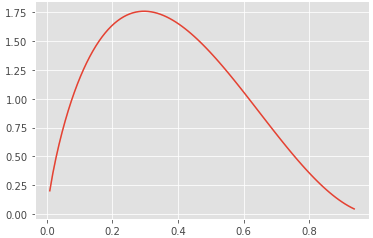#### 棄却サンプリングの実行

では、いよいよ棄却サンプリングを試してみます。

まずは定数を求める必要があるのですが、下の図からわかるようにはベータ分布の最大値と求めることが出来ます。

そして、横軸方向について一様分布からランダムな値を決めます。具体的には0から1の間で一つ値を決定することになります。

この処理によって横軸と縦軸のスケールの異なる一様分布から生成された二つの乱数によって座標が一点決まることになります。

そしてこの乱数によって求まった値が予測分布よりも下の範囲であれば採用します。とてもシンプルですね！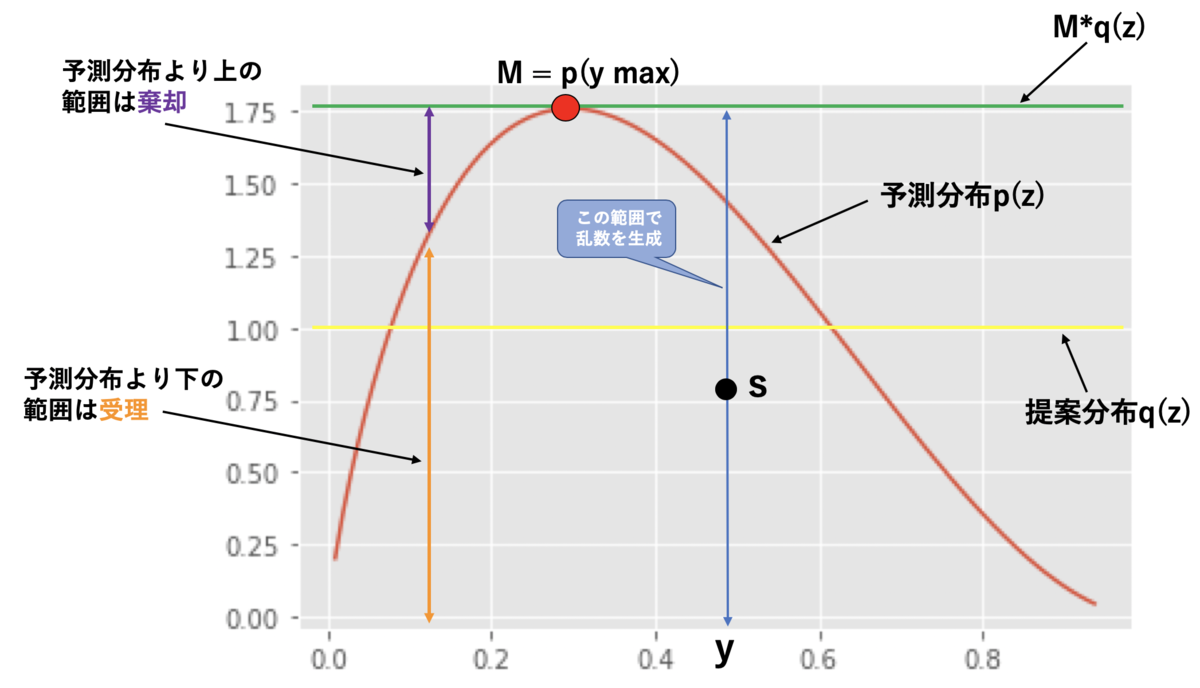では、実装して結果を見ていきます。

まずはを求めますが、今回はScipyのOptimize関数を使います。ScipyのOptimize関数は目的関数を最適化（最小値もしくは最大値を求める）する便利な関数です。

f = beta(a=a, b=b).pdf
res = opt.fmin(lambda x: -f(x), 0.1)
M = f(res)
print("M : ", M)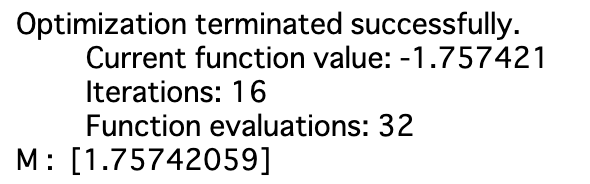にマイナスをつけているのは、ベータ分布の最大値を求めるために最小値問題に帰着させるためです。

まずは乱数を1000個生成した場合を見てみます。

N_MC = 1000

y = uniform.rvs(size=N_MC)
mq = M * uniform.rvs(size=N_MC)
accept = y[mq <= f(y)]

plt.hist(accept, normed=True, bins=35, rwidth=0.8, label="rejection sampling")
x = np.linspace(beta.ppf(0.001, a, b), beta.ppf(0.999, a, b), 100)
plt.plot(x, beta.pdf(x, a, b), label="target distribution")
plt.legend()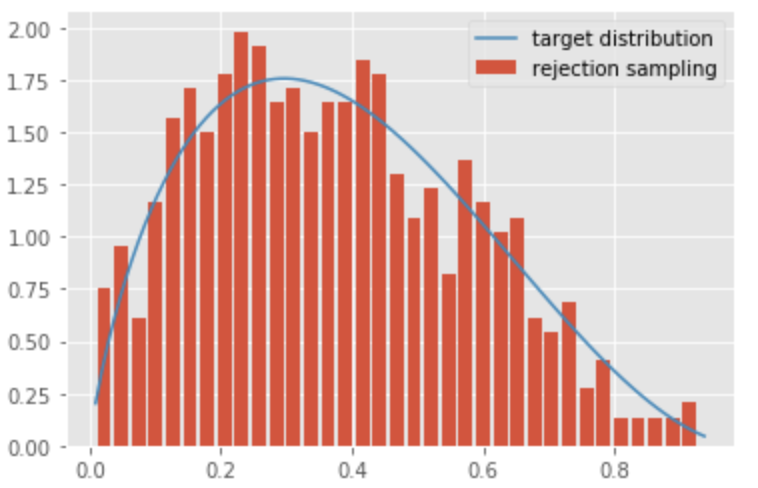1000個の乱数だと、まだ少し予測分布（ベータ分布）に対して少しバラツキがありますね。

では、乱数を50000個に増やしてみましょう。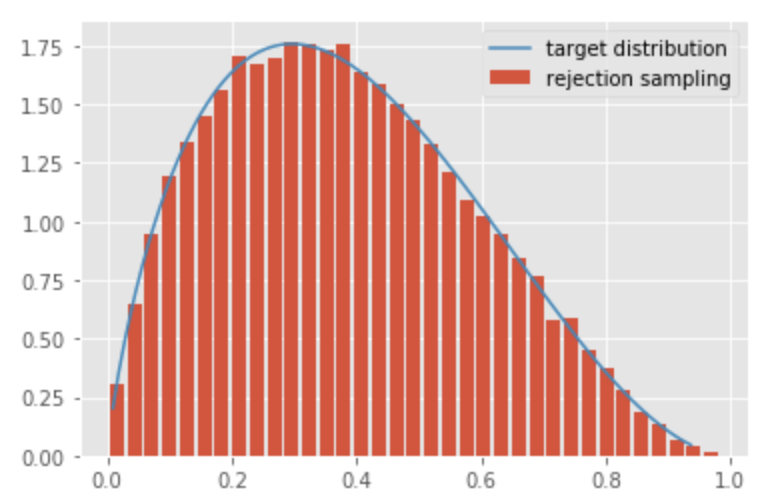すると、乱数が1000個に比べてベータ分布に対してほぼ同じような曲線を描いていることがわかります。

# Test Driving the Proposed Vue.js Function API

By Robin Dickson, software engineer at UniFa.

An RFC (request for comments) for Vue.js was published that explains the plan for a new Function API. Following that, a plugin was created that allows the proposed Function API to be used in current Vue applications: vue-function-api.

I thought I would experiment with the Function API by building a mini app.

## Function API Installation

The base app was created using vue cli, and the vue-function-api plugin installed using yarn:

$vue create janken$ yarn add vue-function-api

Then the plugin installed explicitly:

import Vue from 'vue'
import { plugin } from 'vue-function-api'

Vue.use(plugin)


The current API (Standard API) still works as usual, and it is even possible to use a hybrid approach (Function API + Standard API).

The app I decided to build was Janken, or in English: Rock, Scissors, Paper.

In this app there are 4 features:

• The player can choose their hand
• The computer chooses their hand and a winner is calculated
• The total amount of points (wins) for each player are shown
• The player can change their name

The initial design was:

<template>
<div>
<div class="score">
<div>Player</div>
<div>0 - 0</div>
<div>Computer</div>
</div>
<div class="player-hands">
<div>✊</div>
<div>✊</div>
</div>
<ul class="hand-choices">
<li>✊</li>
<li>✌️</li>
<li>🖐️</li>
</ul>
</div>
</template>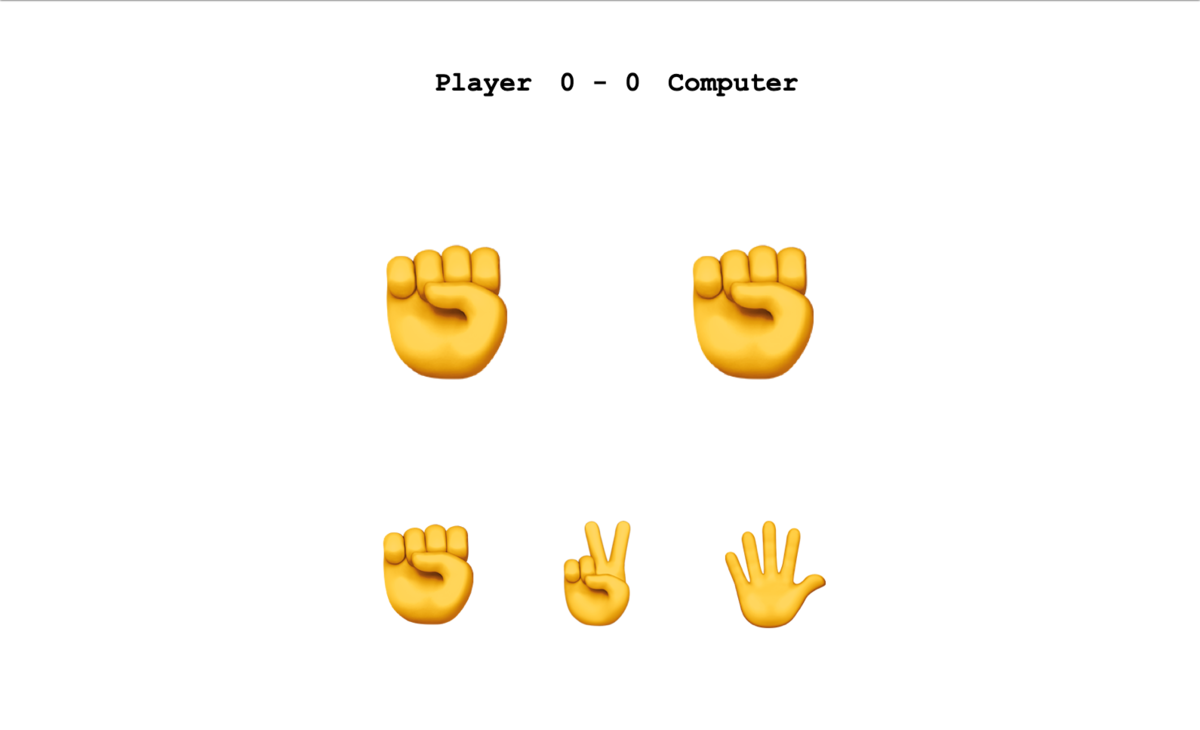## Vue Implementation

### Setup, Data and Value

The first change from the Standard API is that a setup option is used to set up the component logic. If you need to use props they are passed to setup as an argument (more info).

The score data would have previously be stored in the data option, which is not used in the Function API. Instead the data is stored by using the value API. Data and functions that are used in the template are returned from the setup option.

import { value } from "vue-function-api";

export default {
setup() {
const playerScore = value(0);
const computerScore = value(0);

return {
playerScore,
computerScore
};
}
};

<div class="score">
<div>Player</div>
<div>{{ playerScore }} - {{ computerScore }}</div>
<div>Computer</div>
</div>


### Methods

The next task was to enable the player to choose their hand. Using the Standard API this can be done using the methods option. In the Function API the same can be done using a function.

export default {
setup() {
// ...
const playerHand = value(null);

function submitHand(hand) {
playerHand.value = hand;
}
return {
playerScore,
computerScore,
playerHand,
submitHand
};
}
};


To set (and also get) the value of playerHand within setup playerHand.value must be used.

### Computed

The hands are displayed in the UI using emoji. The hand data stored as a string ('rock') is converted to an emoji ('✊') with the computed API (similar to the Standard API's computed option). Again this is stored to a variable and returned from setup to be used in the template.

import { value, computed } from "vue-function-api";

export default {
setup() {
const handsToEmoji = {
rock: "✊",
scissors: "✌️",
paper: "🖐️"
};

const isShowGameHands = value(false);
// ...
const playerHand = value(null);

// ...
const playerDisplayEmoji = computed(() =>
isShowGameHands.value
? handsToEmoji[playerHand.value]
: handsToEmoji["rock"]
);
// ...
return {
// ...
playerDisplayEmoji,
computerDisplayEmoji
};
}
};


If there are many methods or computed values in the returned object, these can be grouped into a single object and destructured in the object returned from setup.

// example code (not from to the Janken app)
const methods = {
methodA() {
// ...
},
methodB(arg) {
// ...
}
}

const computeds = {
computedA: computed(() => 'a'),
computedB: computed(() => 'b')
};

return {
...computeds,
...methods
};


(see in this example)

### Composition Functions

After adding the logic for the game and editing the player name I tried refactoring using a technique made possible in the Function API. Using a composition function the logic (variables and methods) could be extracted to a separate function, and then included in the object returned from the main setup option.

function useName() {
const playerName = value("Player");
const isEditingName = value(false);

function editName() {
isEditingName.value = true;
}
function submitName() {
isEditingName.value = false;
}
return { playerName, isEditingName, editName, submitName };
}

export default {
setup() {
// ...

return {
isShowGameHands,
playerScore,
computerScore,
playerHand,
computerHand,
submitHand,
...computeds,
...useName()
};
}
}


By doing this the code can be organised more clearly, collecting related code together rather than it being separated between different options(data, computed, methods, etc) which can happen in the Standard API. It is also possible to reuse the logic in other components.

Although not used in this app lifecycle hooks and watchers are used in a similar way to value and computed and can also be extracted.

The Janken app and code can be seen and used below. It only took a few steps to get started with the Function API, and there are various features I did not use that I'm looking forward to trying. For more information check out the RFC and try it yourself!

<html>
<script src="https://cdn.jsdelivr.net/npm/vue/dist/vue.js"></script>
<script src="https://unpkg.com/vue-function-api@1.0.4/dist/vue-function-api.umd.js"></script>
<title></title>
<body>
<div id="app">
<main>
<div>
<div v-if="isEditingName" class="emoji-button" @click="submitName">🆗</div>
<div v-else class="emoji-button" @click="editName">✏️</div>

<div class="score">
<div class="input-wrapper" v-if="isEditingName">
<input v-model="playerName" @keyup.enter="submitName" type="text" maxlength="7" />
</div>
<div v-else>{{ playerName }}</div>
<div>{{ playerScore }} - {{ computerScore }}</div>
<div>Computer</div>
</div>
<div class="player-hands">
<div>{{ playerDisplayEmoji }}</div>
<div>{{ computerDisplayEmoji }}</div>
</div>
<ul class="hand-choices">
<li :class="{ selected: playerHand === 'rock' }" @click="sumbmitHand('rock')">✊</li>
<li :class="{ selected: playerHand === 'scissors' }" @click="sumbmitHand('scissors')">✌️</li>
<li :class="{ selected: playerHand === 'paper' }" @click="sumbmitHand('paper')">🖐️</li>
</ul>
</div>
</main>
</div>
</body>
</html>

main {
font-family: "Courier New", Courier, monospace;
width: 280px;
margin: auto;
}
.score {
display: flex;
justify-content: space-between;
margin-top: 40px;
font-size: 18px;
font-weight: bold;
}
.score div:nth-child(1) {
width: 35%;
text-align: right;
}
.score div:nth-child(2) {
width: 30%;
text-align: center;
}
.score div:nth-child(3) {
width: 35%;
text-align: left;
}
.player-hands {
display: flex;
justify-content: space-between;
margin-top: 80px;
font-size: 20vh;
}
.hand-choices {
display: flex;
justify-content: space-between;
margin-top: 60px;
}
.hand-choices li {
font-size: 15vh;
list-style: none;
cursor: pointer;
}
.hand-choices li.selected {
text-decoration: lightblue underline;
transition: font-size 0.2s;
}
.score div {
}
.score .input-wrapper {
}
input[type="text"] {
width: 80px;
margin: 0 0 0 15px;
border: 2px solid #ccc;
font-family: "Courier New", Courier, monospace;
}
.emoji-button {
position: fixed;
top: 10px;
left: 10px;
cursor: pointer;
}
const { plugin, value, computed } = vueFunctionApi;

Vue.config.productionTip = false;
Vue.use(plugin);

function useName() {
const playerName = value("Player");
const isEditingName = value(false);
function editName() {
isEditingName.value = true;
}
function submitName() {
isEditingName.value = false;
}
return { playerName, isEditingName, editName, submitName };
}

var app = new Vue({
el: '#app',
setup() {
const handsToEmoji = { rock: "✊", scissors: "✌️", paper: "🖐️" };
const isShowGameHands = value(false);
const playerScore = value(0);
const computerScore = value(0);
const playerHand = value(null);
const computerHand = value(null);
const computeds = {
playerDisplayEmoji: computed(() =>
isShowGameHands.value
? handsToEmoji[playerHand.value]
: handsToEmoji["rock"]
),
computerDisplayEmoji: computed(() =>
isShowGameHands.value
? handsToEmoji[computerHand.value]
: handsToEmoji["rock"]
)
};
function sumbmitHand(hand) {
playerHand.value = hand;
runGame();
}
function randomHand() {
const hands = Object.keys(handsToEmoji);
return hands[Math.floor(Math.random() * hands.length)];
}
const handToWeakness = {
rock: "paper",
scissors: "rock",
paper: "scissors"
};
if (handToWeakness[computerHand.value] === playerHand.value) {
playerScore.value++;
} else if (handToWeakness[playerHand.value] === computerHand.value) {
computerScore.value++;
}
}
function runGame() {
computerHand.value = randomHand();
isShowGameHands.value = true;
}
return {
isShowGameHands,
playerScore,
computerScore,
playerHand,
computerHand,
sumbmitHand,
...computeds,
...useName()
};
}
}).\$mount('#app')



# 赤ちゃん１人とルーター２つ

こんにちは。サーバーサイドエンジニアの柿本です。

ユニファではリモートワークを取り入れていますが、自宅で作業を行うにはそれなりの環境が必要となります。 私は自宅のリビングが好きなので、リモートワークの時はいつもリビングで作業しています。

しかし、寝室には電波が届きません！我が家はびっくりするくらい狭いのですが、NTTから貸し出されているルーター（ONUと一体型のもの）の電波はさらにびっくりするくらい弱いのです！そのため、電波が強めのルーターをAPとして使うために買いました。

しかし、この春から子供が保育園に通い始めたために寝室に移動する必要がなくなり、ルーターは黒光りするただの飾りものとなってしまいました。

## やること

ググってみると手順はすごく簡単で、

1. DD-WRTをサイトからダウンロード
2. ルーターの管理画面( http://192.168.11.1 )にアクセス
3. ファームウェアの更新メニューからファイルを選んで更新
4. 『dd-wrt』というSSIDに接続
5. ルーターの管理画面( http://192.168.1.1 )に接続
6. 諸々の設定

といった感じです。

### 1. DD-WRTのダウンロード

DD-WRTのサイト( https://dd-wrt.com )のRouter Databasesから「WZR-HP-AG300H」で検索すればすぐに見つかりました。

### 2. ルーターの管理画面( http://192.168.11.1 )にアクセス

http://192.168.11.1 で管理画面が開くはずですが、なぜかアクセスできません。。。pingも通りません。。。

MACアドレスがわからないので順番にアクセスを試してみると「192.168.1.8」がBuffaloのルーターでした！ よくよく考えるとNTTから貸し出されているルーターでルーティングしているので、Buffaloのルーターが想定と違うIPでもそりゃそうか、という感じですね。

APとして使っている場合はハマりポイントかもしれません。

### 4. 『dd-wrt』というSSIDに接続

10分ほどドキドキする時間が続きましたが、「dd-wrt」というSSIDが出てきたのでひとまず安心です！

### 5. ルーターの管理画面( http://192.168.1.1 )に接続

パスワード設定画面のあと、管理画面に無事に到達しました。

### 6. 諸々の設定

#### APとして使う設定

「Apply Setting」を押すとルーターの再起動がかかりますが、再起動後は設定画面が http://192.168.1.100 になるため、注意が必要です。

#### SSIDとWPA

「WZR-HP-AG300H」というデバイスは2.4GHzと5GHzで通信できますが、初期状態だとどちらも「dd-wrt」というSSIDになるので見分けがつきません。 なので、それぞれ別のSSIDを設定してあげます。

このままだとご近所さんにフリーWi-Fiを提供して我が家を危険に晒してしまうので、WPAの設定も行います。

#### ssh接続

せっかくLinuxマシーンを手に入れたので、ssh接続できなくてはつまらない！ということで設定します。

「Apply Setting」を押してしばらくすると、ssh接続できました！

## まとめ

ルーターの管理画面のアドレスがコロコロ変わるので迷子になりかけますが、そこさえ突破できれば、ただのルーターがちょっとしたLinuxサーバーに変身です！

UniFaでは使わなくなった電子機器を改造せずにいられないエンジニアを募集中です！ご応募お待ちしております！

# Experimental method for Bio-Data augmentation using only two observations for deep learning applications.

By Matthew Millar R&D Scientist at ユニファ

This blog will show a new experimental method for data augmentation geared towards bio-science for deep learning. This is important for several reasons. 1: Collecting data is time-consuming especially in collecting large enough observations for training deep learning models. 2: It can be difficult to collect or sample enough observations due to the lack of access or chances to make collections. 3: Collecting observations can only be done at certain times or during certain periods, or the period of time for sampling has passed so the collection of further/more observations are impossible. 4: There are few species available to collect samples from. These are just 4 simple reasons why data augmentation is needed for biological studies.

### Methods for Data Augmentation

The simplest method for data augmentation is to match the generated data both statistically and logically to the observed data. This means that the data that is generated should have a similar look and feel of the real-world data. The two data sets should have similar distributions, mean, modes, etc. to ensure that the data truly simulates the observed sequences. The simulated data should also be logically like the data that is observed. This means that the simulated data should not have outliers model into it as this will confuse any model. The augmented data should flow alongside the observations and almost mirror each observation. But, just copying the real observations is not an appropriate method for data augmentation. The observations should change slightly. For example, common methods for data augmentations in CNN are image rotation, flipping, cropping, changing color, etc. to create “new” unseen images for a CNN to be trained on. This is also true for numerical data, but not as easy as just flipping the numbers from 10 to 01 as they are not the same.

There are very few methods that exist for data augmentation for numerical data. There are even fewer geared specifically towards biodata or biostudies. This blog will show a new method for generating near-infinite observations based simply on the minimum and maximum observations in a data set.
The data set that I am using is a publicly available data set of Body Measurements (BDIMS)(Heinz, Peterson, Johnson, & Kerk, 2003). This data set is the girth and skeletal measurement of 247 men and 260 women.

Now let's get into the coding aspect of it:

### CODE

First, let's get all the import statements out of the way.

import numpy as np
import pandas as pd
%matplotlib inline
import matplotlib.pyplot as plt
import pymc3 as pm
import theano
from statsmodels.formula.api import glm as glm_sm
import statsmodels.api as sm
from pandas.plotting import scatter_matrix
from random import randint


Next, we need to do some quick examination of the data we downloaded.

# Read the data in from the csv file
print(data.columns)

Index(['bia.di', 'bii.di', 'bit.di', 'che.de', 'che.di', 'elb.di', 'wri.di',
'kne.di', 'ank.di', 'sho.gi', 'che.gi', 'wai.gi', 'nav.gi', 'hip.gi',
'thi.gi', 'bic.gi', 'for.gi', 'kne.gi', 'cal.gi', 'ank.gi', 'wri.gi',
'age', 'wgt', 'hgt', 'sex'],
dtype='object')

Now we know the colum names. Lets get rid of some of the data we dont want to make it simpler and easier to use.

filter_data = data.filter(['sex','hgt','wgt', 'che.gi','hip.gi', 'kne.gi','thi.gi', 'ank.gi', 'wri.gi', 'wai.gi' ], axis=1)

   sex    hgt   wgt  che.gi  hip.gi  kne.gi  thi.gi  ank.gi  wri.gi  wai.gi
0    1  174.0  65.6    89.5    93.5    34.5    51.5    23.5    16.5    71.5
1    1  175.3  71.8    97.0    94.8    36.5    51.5    24.5    17.0    79.0
2    1  193.5  80.7    97.5    95.0    37.0    57.3    21.9    16.9    83.2
3    1  186.5  72.6    97.0    94.0    37.0    53.0    23.0    16.6    77.8
4    1  187.2  78.8    97.5    98.5    37.7    55.4    24.4    18.0    80.0

Much nicer. Now we only want to look at one subject as this is biological data. So we will filter out females from males and just look at males. This process will work on both sexes as the steps will be the same, but doing both at the same time will yield poor results as there are biological differences between males and females in general.

# Split between male and female
# After sperating the two exes lets drop the sex collumn as we dont need it
male = male.drop(['sex'], axis=1)
male.describe()

hgt	wgt	che.gi	hip.gi	kne.gi	thi.gi	ank.gi	wri.gi	wai.gi
count	247.000000	247.000000	247.000000	247.000000	247.000000	247.000000	247.000000	247.000000	247.000000
mean	177.745344	78.144534	100.989879	97.763158	37.195547	56.497976	23.159109	17.190283	84.533198
std	7.183629	10.512890	7.209018	6.228043	2.272999	4.246667	1.729088	0.907997	8.782241
min	157.200000	53.900000	79.300000	81.500000	31.100000	46.800000	16.400000	14.600000	67.100000
25%	172.900000	70.950000	95.950000	93.250000	35.750000	53.700000	22.000000	16.500000	77.900000
50%	177.800000	77.300000	101.000000	97.400000	37.000000	56.000000	23.000000	17.100000	83.400000
75%	182.650000	85.500000	106.050000	101.550000	38.450000	59.150000	24.300000	17.850000	90.000000
max	198.100000	116.400000	118.700000	118.700000	45.700000	70.000000	29.300000	19.600000	113.200000


Now with the first step of preprocessing, we can get into the process of creating the dataset from only two points! These two points will be the minimum and maximum based on height. Height is chosen because this variable is the dominating variable in biology and bio-mass. Weight is normally heavily dependant on height (pun intended). The dependent variable will be weight. (X = height Y = weight).
So let's find the smallest and largest person in the dataset.

# Find the smallest item based on height
# Create a new dataframe of the smallest and larget
min_max_male = pd.DataFrame(male[male.hgt == male.hgt.max()])
min_max_male = min_max_male.append(male[male.hgt == male.hgt.min()])
# Sort by height
sort_min_mix_male = min_max_male.sort_values('hgt')
print(sort_min_mix_male)
hgt   wgt  che.gi  hip.gi  kne.gi  thi.gi  ank.gi  wri.gi  wai.gi
105  157.2  58.4    91.6    91.3    35.5    55.0    20.8    16.4    80.6
126  198.1  85.5    96.9    94.9    39.2    54.4    27.5    17.9    82.5
ax1 = min_max_male.plot.scatter(x='hgt',y='wgt',c='DarkBlue')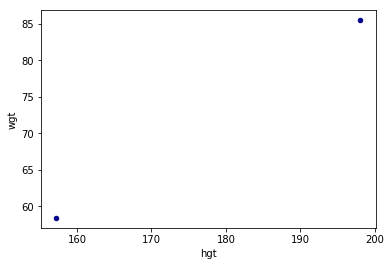Max Min PlotSo the first and simplest method to interpolation is linear regersion. This will give us a few extra points of missing data.

# Now use linear regression to fill in some of the missing points
import numpy as np
from sklearn.linear_model import LinearRegression
x = np.array([min_max_male.hgt.min(),min_max_male.hgt.max()]).reshape((-1, 1))
y = np.array([min_max_male.wgt.min(), min_max_male.wgt.max()])
# Define a linear regerssion model
model = LinearRegression()
model.fit(x, y)
r_sq = model.score(x, y)
print('coefficient of determination:', r_sq)
print('intercept:', model.intercept_)
print('slope:', model.coef_)

coefficient of determination: 1.0
intercept: -45.75941320293397
slope: [0.66259169]

Now to make new points.

prediction = []
gen_height = []
for i in range(int(min_max_male.hgt.min()), int(min_max_male.hgt.max())):
new_x = np.array(i).reshape((-1, 1))
gen_height.append(i)
pred = model.predict(new_x)
prediction.append(pred)

print(len(prediction))
print(len(gen_height))
print(prediction)
print(gen_height)
41
41
58.267481662591706
157
# Lets plot the results
import matplotlib.pyplot as plt

old_min_hgt = min_max_male.hgt.min()
old_max_hgt = min_max_male.hgt.max()
old_min_wgt = min_max_male.wgt.min()
old_max_wgt = min_max_male.wgt.max()

plt.plot(gen_height, prediction, 'ro')
plt.plot(old_min_hgt, old_min_wgt, 'bo')
plt.plot(old_max_hgt, old_max_wgt, 'bo')
plt.show()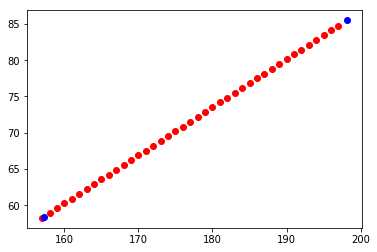Linear RegressionOk looks fine so far. The blue dots are the original data (min and max) and the red dots are the newly generated data. This makes sense as weight should increase as height increases. But, not really. There are variations in weight because of other factors. Also, 41 new points don't make a deep learning set.
Lets create a few more points:

# Now lets fine tune the hieght veriable by a float instead of a int
# We can resue the linerar regression model to generate more data
# Go from 41 observations to 409000 observatsions
# All equally possible to occure in the real world
current_hgt = min_max_male.hgt.min()
count = 0
large_hgt = []
while current_hgt <= min_max_male.hgt.max():
# increase the height by 0.1 cm
current_hgt +=0.0001
large_hgt.append(current_hgt)
count +=1
print(len(large_hgt))

409000

# Now using the newlly generated fine scale height lets get the weight
large_pred = []
for h in large_hgt:
new_x = np.array(h).reshape((-1, 1))
pred = model.predict(new_x)
large_pred.append(pred)

print(len(large_pred))

409000
# Now lest plot everything again

plt.plot(large_hgt, large_pred, 'go')
plt.plot(gen_height, prediction, 'ro')
plt.plot(old_min_hgt, old_min_wgt, 'bo')
plt.plot(old_max_hgt, old_max_wgt, 'bo')
plt.show()


As you can see perfectly overlaps and each observation makes sense and is logical.
The blue dots are the original, the red is the first step, and the green is fine-tuned steps.
This jumps from 2 observations (min and max) to 41 observations (fully synthetic) to 409000 observations.
But in the real world, biology does not always follow a linear line
Let's introduce some variability into the data generation!

# Define a new line using all the data from the real data set
# Define a linear regerssion model
X = np.array(male.hgt).reshape(-1, 1)
Y = np.array(male.wgt).reshape(-1, 1)

model2 = LinearRegression()
model2.fit(X,Y)
r_sq2 = model2.score(X,Y)
print('coefficient of determination:', r_sq2)
print('intercept:', model2.intercept_)
print('slope:', model2.coef_)

coefficient of determination: 0.28594874074704446
intercept: [-60.95336414]
slope: [[0.78256845]]
# Linear regresion using real data
y_pred = model2.predict(X)
# Now plot all the data
plt.plot(X, y_pred, color='blue', linewidth=3)
plt.plot(male.hgt, male.wgt, 'yo')
plt.plot(large_hgt, large_pred, 'go')
plt.plot(gen_height, prediction, 'ro')
plt.plot(old_min_hgt, old_min_wgt, 'bo')
plt.plot(old_max_hgt, old_max_wgt, 'bo')
plt.show()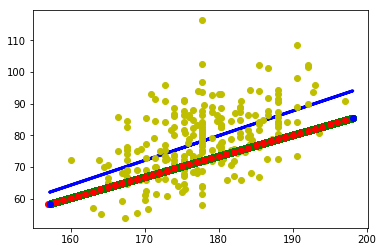Real DataAs you can see the regression line is some what close to the line of data that is generated. It is not perfect and there will be a lot of variability between the two datasets. But seeing that this is only based on two observations, (the min and max) the lines are pretty close. The intercept and slope are close enough to use the ones found from the two points only. So let us continue and make a fully synthetic deep learning dataset from two observations.

# The slope of the line is b, and a is the intercept found from Sklenar linear model
# Simple Linear regressoin model Y = a + bX that will be the model for out MCMC
alpha =  -45.75941320293397 # Intercept
beta = [0.66259169] # Slope
X = np.array(large_hgt)
Y = np.array(large_pred)
print(len(X))
print(len(Y))

409000
409000

# Weight Histogram
hist = male.hist(column='wgt')


#Normal distribution. mu is the mean, and sigma is the standard deviation.
# Seeing that the weight is normally distributed (basically) we can use that knowledge to generate new data via a normally
# Distrubuted method

#for random.normalvariate(mu, sigma)
std = np.std(X, axis=0)
real_std = np.std(male.wgt, axis=0)
print(std)
print(real_std)
11.806813005284504
10.491587167890629

temp_min_max = []
temp_min_max.append(male.wgt.max())
temp_min_max.append(male.wgt.min())
mean = np.mean(temp_min_max)
real_mean = np.mean(male.wgt)
print(mean)
print(real_mean)
85.15
78.14453441295547


Looking at the mean and standard deviation they are close enough for this example. Lets make a Million data points for our new dataset! That should be enough for any deep learning dataset.

new_X = []
new_Y = []
for i in range(0,1000000):
index = randint(0, len(X) -1)
new_X.append(X[index])
new_Y.append(np.random.normal(mean,std))
plt.plot(new_X, new_Y, 'go',marker='^')
plt.plot(male.hgt, male.wgt, 'yo')
plt.plot(large_hgt, large_pred, 'go')
plt.plot(gen_height, prediction, 'ro')
plt.plot(old_min_hgt, old_min_wgt, 'bo')
plt.plot(old_max_hgt, old_max_wgt, 'bo')
plt.show()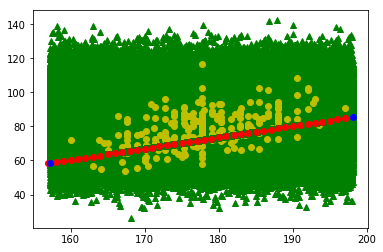A Million Data points!Well thats no good. Now to be fair, given a infinate number of samples, it is highly likely that at least for each point there would have been someone that mathces the height and weight on this chart, but that is like using a shotgun to fish. It is not as accurate and not really following the regression line of the real data which means that the dataset is not useful and cannot be used in a deep learning model as it wont learn anything.
So how can we fix this?
Let's perform some rejections by using a concept of banding. So if the observation falls outside the bands it won't get plotted. The bands themselves set up an upper and lower limit so that all predictions will have to fall within these limits. To form these limit expert knowledge of the observed phenomenon is needed especially for only two observations, luckily for us, we have more than two observations so we can define out limits based on the full real dataset.

# Use upper and lower limits to reject samples
def make_sample(lower, upper, mean, std):
sample = np.random.normal(mean,std)
if lower < sample < upper:
return sample
else:
make_sample(lower, upper, mean, std)

# Define bands for each interval
# The more bands the finer the level of rejection
# Each item in the array is defined as
# [band lower, band upper, lower limit, upper limit]
band1 = [0, 155, 50, 70]
band2 = [156,160, 55, 70]
band3 = [161, 165, 56, 75]
band4 = [166, 170, 57, 80]
band5 = [171, 175, 60, 88]
band6 = [176, 180, 60, 94]
band7 = [181, 185, 60, 100]
band8 = [186, 190, 63, 105]
band9 = [191, 195, 64, 110]
band10 = [196, 299, 65, 110]
# Put all the bands into a single array for easy use
bands = []
bands.append(band1)
bands.append(band2)
bands.append(band3)
bands.append(band4)
bands.append(band5)
bands.append(band6)
bands.append(band7)
bands.append(band8)
bands.append(band9)
bands.append(band10)

new_X = []
new_Y = []
for i in range(0, 1000000):
index = randint(0, len(X) -1)
for band in bands:
if band <= X[index] <= band:
new_X.append(X[index])
new_Y.append(make_sample(band, band, mean, std))

plt.plot(new_X, new_Y, 'go',marker='^')
plt.plot(male.hgt, male.wgt, 'yo')
plt.plot(large_hgt, large_pred, 'go')
plt.plot(gen_height, prediction, 'ro')
plt.plot(old_min_hgt, old_min_wgt, 'bo')
plt.plot(old_max_hgt, old_max_wgt, 'bo')
plt.show()


Which gives us this!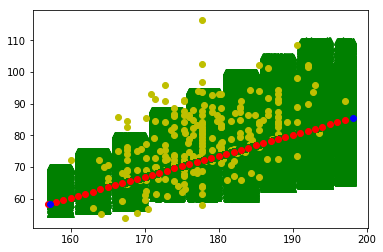Banded Data There are still a million points, but some my be repeated. But, the general flow is far more similar to the real data which is perfect now for training a deep learning model.

### Conclusion

From this blog, we saw how to use only two observations, the minimum and maximum, and how to create a fully synthetic dataset that can be used for deep learning.
The main idea when building a fully synthetic dataset is to ensure it is statistically and logically similar to that of the observed/real dataset. This gives the benefit of creating a large training dataset and then using the real data as a testing set. This can give very good results when creating a deep learning model as you won't have to train the model on the very limited (and precious) real data that can be very difficult to capture or collect.

This approach can be improved significantly, especially in the banding section. By adding a larger number of bands, smoothing out the lower and upper limits, and even using more complex algorithms like a random walk can improve the final results. But, this method still needs to be vetted before use in different models and/or real-world applications. The next step would be to model more independent variables, other phenomenons, and improve the generation steps.

### References:

Heinz G, Peterson LJ, Johnson RW, Kerk CJ. 2003. Exploring Relationships in Body Dimensions. Journal of Statistics Education 11(2).

# ひらがな練習アプリを作ってみる

こんにちは。iOSエンジニアのキムです。ユニファの開発ブログでは初の投稿になります。よろしくお願いします。

### 処理内容

iOSでは指で画面をなぞることでタッチイベントが発生します。 今回はタッチイベント発生時にUIBezierPathを使って線を描画します。 以下のような流れになります。

touchesBegan(_:with:) タッチ開始時

override func touchesBegan(_ touches: Set<UITouch>, with event: UIEvent?) {
if let touch = touches.first?.location(in: self) {
path = UIBezierPath()
path?.lineWidth = 30
path?.lineCapStyle = .round
path?.lineJoinStyle = .round
path?.move(to: touch)
}
}


touchesMoved(_:with:) タッチしたまま指を移動

override func touchesMoved(_ touches: Set<UITouch>, with event: UIEvent?) {
if let touch = touches.first?.location(in: self) {
setNeedsDisplay()
}
}


touchesEnded(_:with:) タッチした指が離れる

override func touchesEnded(_ touches: Set<UITouch>, with event: UIEvent?) {
if let touch = touches.first?.location(in: self) {
setNeedsDisplay()
}
}


AVSpeechSynthesizerを使ってテキストを読み上げる処理

func speak(string: String) {
self.speechSynthesizer = AVSpeechSynthesizer()
let utterance = AVSpeechUtterance(string: string)
utterance.voice = AVSpeechSynthesisVoice(language: "ja-JP")
utterance.rate = AVSpeechUtteranceMinimumSpeechRate
utterance.pitchMultiplier = 1
self.speechSynthesizer.speak(utterance)
}

// 利用方法
speak(string: "あ")


# Go 言語で AWS S3 のダウンロードに時間がかかったとき中断する

こんにちは、 rightgo09 です。